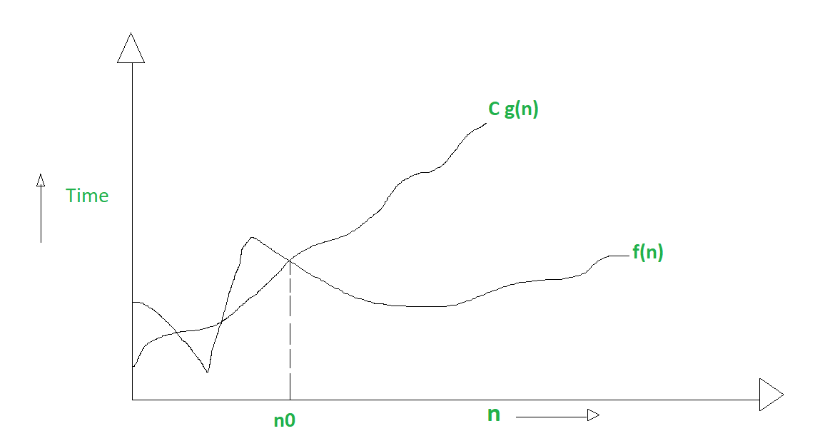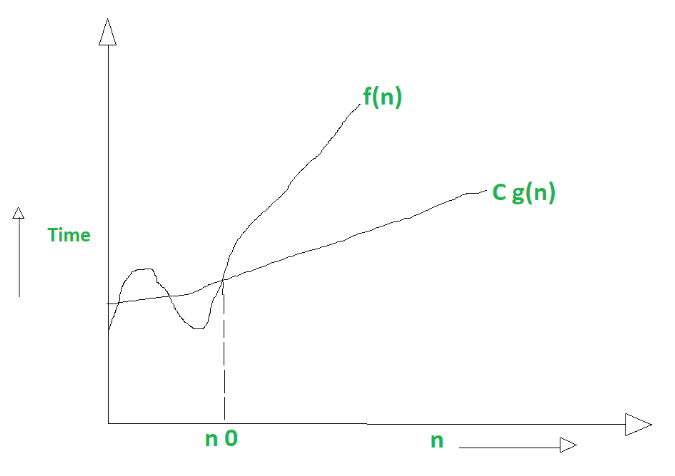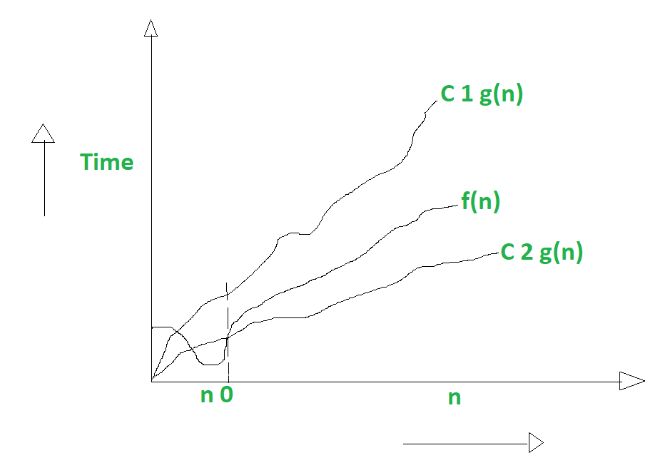# Difference between Big Oh, Big Omega and Big Theta

Prerequisite – Asymptotic Notations, Properties of Asymptotic Notations
1. Big Oh notation (O) :
Big oh notation is used to describe asymptotic upper bound.

Mathematically, if f(n) describe running time of an algorithm; f(n) is O(g(n)) if there exist positive constant C and no such that

`0 <=f(n) <= c g(n) for all n>=n0`

n = used to give upper bound an a function.
If a function is O(n), it is automatically O(n-square) as well !

Graphic example for Big oh (O) :2. Big Omega notation (Ω) :
Just like O notation provide an asymptotic upper bound, ? notation provides asymptotic lower bound.
Let f(n) define running time of an algorithm;

f(n) is said to be Ω(g (n)) if there exists positive constant C and (n 0 ) such that

`O<= C g(n) <= f(n) for all n>=n 0`

n= used to given lower bound on a function
If a function is O(n-square ) it is automatically O(n) as well.

Graphical example for Big Omega (Ω) :3. Big Theta notation (Θ) :
Let f(n) define running time of an algorithm.

f(n) is said to be Θ(g(n)) if f(n) is O(g(n)) and f(n) is Ω(g(n))

Mathematically,

```O<=f(n)<=C 1 g(n) for n>=n 0

O<= C 2 g(n)<=f(n) for n >=n 0 ```

Merging both the equation, we get :

`O<=C 2 g(n)<=f(n)<=C 1 g(n) for n>=n 0 `

The equation simply means there exist positive constants C 1 and C 2 such that f(n) is sandwich between C 2 g(n) and C 1 g(n).

Graphic example of Big Theta (Θ) :Difference Between Big oh, Big Omega and Big Theta :

S.No. Big Oh Big Omega Big Theta
1. It is like <=
rate of growth of an algorithm is less than or equal to a specific value
It is like >=
rate of growth is greater than or equal to a specified value
It is like ==
meaning the rate of growth is equal to a specified value
2. The upper bound of algorithm is represented by Big O notation. Only the above function is bounded by Big O. asymptotic upper bond is it given by Big O notation. The algorithm’s lower bound is represented by Omega notation. The asymptotic lower bond is given by Omega notation The bonding of function from above and below is represented by theta notation. The exact asymptotic behavior is done by this theta notation.
3. Big oh (O) – Worst case Big Omega (Ω) – Best case Big Theta (Θ) – Average case
4. Big-O is a measure of the longest amount of time it could possibly take for the algorithm to complete. Big- Ω is take a small amount of time as compare to Big-O it could possibly take for the algorithm to complete. Big- Θ is take very short amount of time as compare to Big-O and Big-? it could possibly take for the algorithm to complete.
5. Mathematically – Big Oh is 0 <=f(n) <= c g(n) for all n>=n0 Mathematically – Big Omega is O<= C g(n) <= f(n) for all n>=n 0 Mathematically – Big Theta is O<=C 2 g(n)<=f(n)<=C 1 g(n) for n>=n 0

Attention reader! Don’t stop learning now. Get hold of all the important DSA concepts with the DSA Self Paced Course at a student-friendly price and become industry ready.

My Personal Notes arrow_drop_upCheck out this Author's contributed articles.

If you like GeeksforGeeks and would like to contribute, you can also write an article using contribute.geeksforgeeks.org or mail your article to contribute@geeksforgeeks.org. See your article appearing on the GeeksforGeeks main page and help other Geeks.

Please Improve this article if you find anything incorrect by clicking on the "Improve Article" button below.

Article Tags :

Be the First to upvote.

Please write to us at contribute@geeksforgeeks.org to report any issue with the above content.